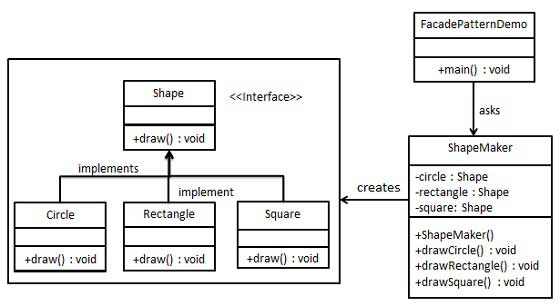# 外观模式

## 实现### 步骤 1

Shape.java

```public interface Shape {
void draw();
}
```

### 步骤 2

Rectangle.java

```public class Rectangle implements Shape {

@Override
public void draw() {
System.out.println("Rectangle::draw()");
}
}
```

Square.java

```public class Square implements Shape {

@Override
public void draw() {
System.out.println("Square::draw()");
}
}
```

Circle.java

```public class Circle implements Shape {

@Override
public void draw() {
System.out.println("Circle::draw()");
}
}
```

### 步骤 3

ShapeMaker.java

```public class ShapeMaker {
private Shape circle;
private Shape rectangle;
private Shape square;

public ShapeMaker() {
circle = new Circle();
rectangle = new Rectangle();
square = new Square();
}

public void drawCircle(){
circle.draw();
}
public void drawRectangle(){
rectangle.draw();
}
public void drawSquare(){
square.draw();
}
}
```

### 步骤 4

```public class FacadePatternDemo {
public static void main(String[] args) {
ShapeMaker shapeMaker = new ShapeMaker();

shapeMaker.drawCircle();
shapeMaker.drawRectangle();
shapeMaker.drawSquare();
}
}
```

### 步骤 5

```Circle::draw()
Rectangle::draw()
Square::draw()
```

App下载